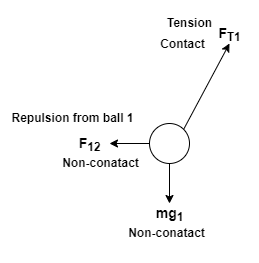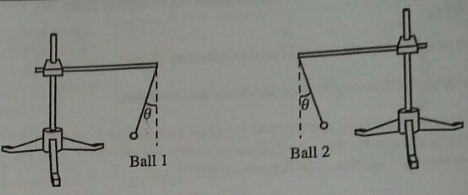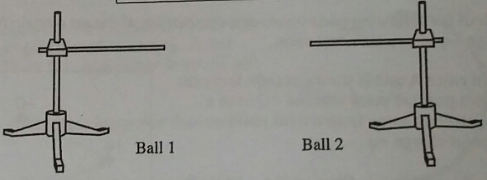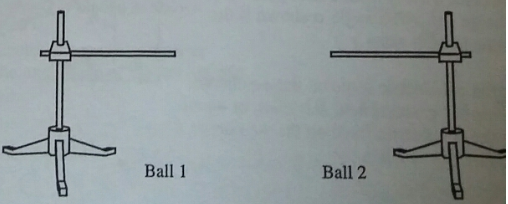# Problem: Two identical metal balls are suspended by insulating threads. Both balls have the same net charge. In this problem, do not assume the balls are point charges a. Draw a separate free-body diagram for each ball. Label the forces to indicate:the object exerting the force,the object on which the force is exerted, the type of force (gravitational, normal, etc.), and whether the force is a contact or a non-contact force.b. Suppose the charge on the second ball is reduced slightly, so that it is less than that on the first ball.Predict whether the angle as that ball 1 makes with the vertical will be greater than, less than or equal to the angle that ball 2 makes with the vertical. Explain. Sketch your answer above.How does the free-body diagram for each ball in this case compare to the corresponding free body diagram that you drew in part a? If the magnitudes or directions of any of the forces change, describe how they change.c. Predict what will happen if the net charge on ball 2 is reduced to zero. Make a sketch to illustrate your answer.

###### FREE Expert Solution

(a)

Like charges repel each other. The two charges repel each other in a horizontal vector. The weight of the balls acts vertically downwards. The tension force acts parallels to the direction of the suspending cord.Free body diagram of ball 1

88% (247 ratings)###### Problem Details

Two identical metal balls are suspended by insulating threads. Both balls have the same net charge. In this problem, do not assume the balls are point charges

a. Draw a separate free-body diagram for each ball. Label the forces to indicate:

• the object exerting the force,
• the object on which the force is exerted,
•  the type of force (gravitational, normal, etc.), and
•  whether the force is a contact or a non-contact force.b. Suppose the charge on the second ball is reduced slightly, so that it is less than that on the first ball.

Predict whether the angle as that ball 1 makes with the vertical will be greater than, less than or equal to the angle that ball 2 makes with the vertical. Explain. Sketch your answer above.

How does the free-body diagram for each ball in this case compare to the corresponding free body diagram that you drew in part a? If the magnitudes or directions of any of the forces change, describe how they change.c. Predict what will happen if the net charge on ball 2 is reduced to zero. Make a sketch to illustrate your answer.